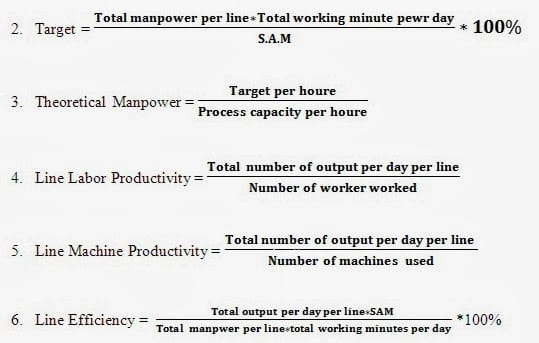# Essential Formulas and Examples for Industrial Engineer in RMG Sector

Last Updated on 15/02/2021

Industrial Engineer in RMG Sector
The ready-made garments and exporting industry is facing heavy challenges due to various factors including global competition, increasing production costs, less productivity or efficiency, labor attrition, etc. Now is a time think to the emerging situation and go deep into analysis of the situation in a realistic manner. We should upgrade the facilities as system run, rather than people run. The industrial engineering (IE) concept needs to be imparted to the facilities to increase productivity in garment industry. In this article I have given some formulas and examples for industrial engineer. I think these formulas and examples will be handful for industrial engineer who are doing job and plan to enter into industrial engineering (IE) department in ready made garment industry.### Some Essential Formulas and Examples for Industrial Engineer

Standard Pitch Time (S.P.T) = Basic Pitch Time (B.P.T) + Allowances (%)7. GSD = (Man power * Work hour) / Target

8. SMV = Basic time + (Basic time * Allowance)

9. Basic time = Observed time * Rating

10. Observed time = Total Cycle time / No of cycle

11. Rating = (Observed Rating * Standard rating) / Standard rating

12. Efficiency = (Earn minute * Available minute) * 100

13. Earn minute = No of Pc’s (Production) * Garments SMV

14. Available minute = Work hour * Manpower

15. Organization Efficiency = (Basic pis time / Bottle neck time) * 100

16. Basic pis time = Total GMT SMV / Total Manpower

17. UCL = Basic pis time / Organization Efficiency

18. LCL = 2 * Basic pis time – UCL

19. Capacity = 60 / Capacity time in minute

20. Cycle Time = 60 / Team target

21. Capacity Achievable = Capacity * Balance

22. Daily output = Work hour / SMV

23. Factory capacity = (Work hour / SMV) * Total worker * Working day * Efficiency

24. CPM = (Total over head cost of the month / No of SMV earners * Work minutes) * Efficiency

25. Required no of operator = Target daily output / Daily output per operator

Examples:

1. Efficiency = Output/Input

= (SMV * Product Quantity) / (Worker * Working Hour * 60)

= [(38.50 * 700) / (60 * 10 * 60)] * 100

= 74% (Line Efficiency)

Here,
SMV=38.50
Working hour=10
No. of worker=60

2. Target/hour = (60/SMV) * Efficiency%

= (60/0.85)*70%

= 50 pcs/hour

Here,
SMV=0.85
Efficiency=70%

3. Target/line = [(No of worker * working hour * 60)/SMV] * Efficiency%

= [(75 * 10 * 60)/23.5] * 60%

= 1150 pcs

Here,
No of workers=75
Working hours=10
SMV=23.5
Efficiency=60%

4. Required days for the schedule = Total order quantity/Average target

= 50000/2000

=25

Here,
Average target=2000
Order quantity=50000

5. CM of garments= (Total Production) / (no of worker * work hour)

= 250000/(1500*10)

=1.66 BDT

Here,
Total Production=250000
No of workers= 1500

6. Factory capacity = [(Work hour * total workers * working day * 60) / SMV] * Efficiency

= [(10 * 1500 * 26 * 60)/14.13] * 55%

= 828025 pcs/month

7. Line GSD/day = (Manpower * work hour) / target

= (50 * 10 * 60)/900

= 33

You may also like: